# the apple and the moon

Physics has been dominated for a very long time by Aristotle's ideas on the subject : Each object has a place of its own, and if we move it from that place, it will do its best to return to it...Everything around us seeks its natural balance. An apple tends to go down, since it is its nature. The Moon orbits the Earth because it is its nature.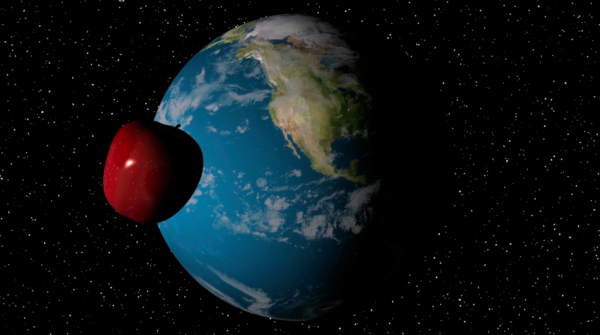In the 17th century, Galileo Galilei (1564-1642) studied falling objects, but it was Isaac Newton  (1643-1727) who formulated the universal law of gravitation: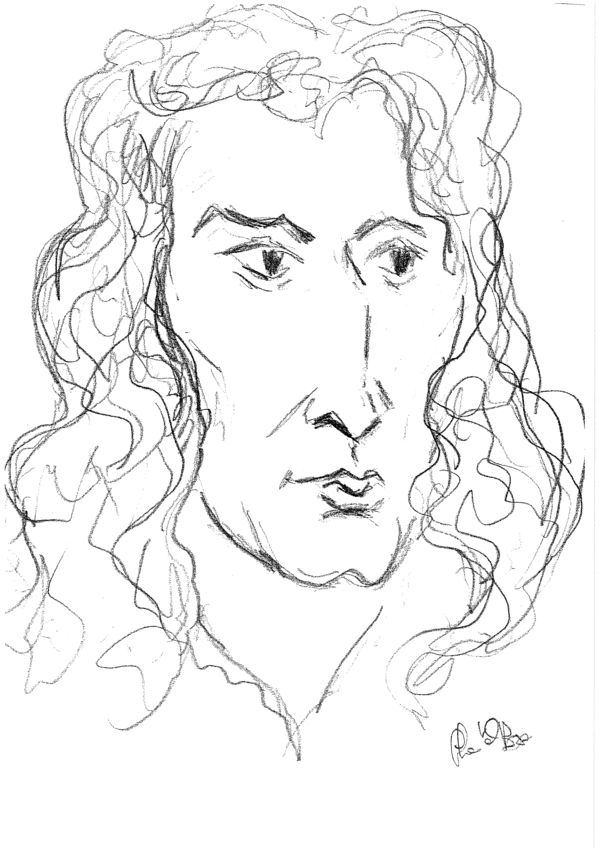« Two bodies attract with a force proportional to each of the masses, and inversely proportional to the square of the distance between them.  »All objects, like apples or the Moon, are attracted by the Earth. It is gravity affecting everything around us. In the vicinity of an apple tree, gravity is nearly constant. When an apple comes loose, gravity does its work and modifies the speed at which the apple falls. Again this is Newton's idea : forces modify speed.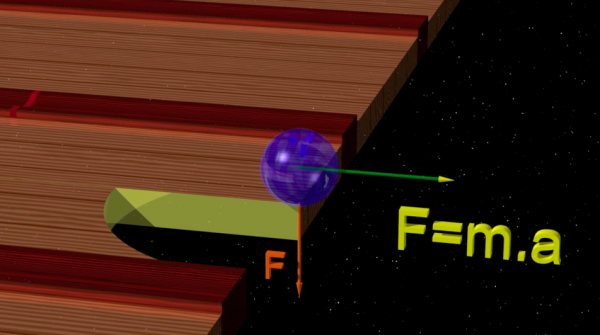Newton goes further and explains how we can calculate the trajectory of an object if we know the forces that act upon it. Newton's formula is one of the most simple, but also most fundamental in physics: F=m.a . Here F is the force, m is the mass and a is the acceleration.  If we know the force and the mass of the object, we also know its acceleration. If on top of that we also know the object's initial position and speed, then Newton's crystal ball lets us predict the future motion of the object in an unambiguous way.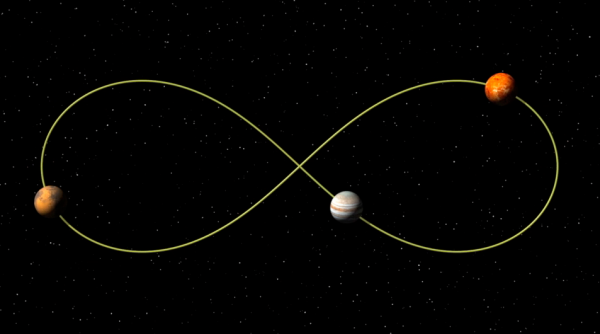Using Newton's formulas it is possible to construct a planetary choreography : three planets with only their mutual attraction acting upon them as forces can be made to travel on the same periodic trajectory. (Provided we can give them the exact starting positions and starting speeds!). Many other such choreographies can be constructed.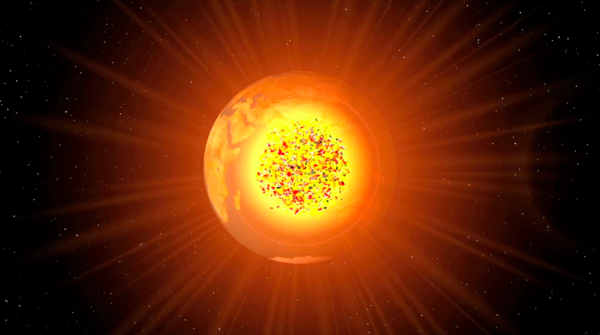As to the question of why the Moon does not fall on the Earth: Newton's answer is that it is indeed falling! If there was no mutual attraction, the Moon would travel in a straight line, ignoring the Earth. However, gravitation bends the Moon's trajectory, so that it is forever 'falling'. The Moon's speed relative to the Earth makes it stay in orbit. Too high a speed and the Moon would disappear forever...Too low a speed and Earth and Moon would collide...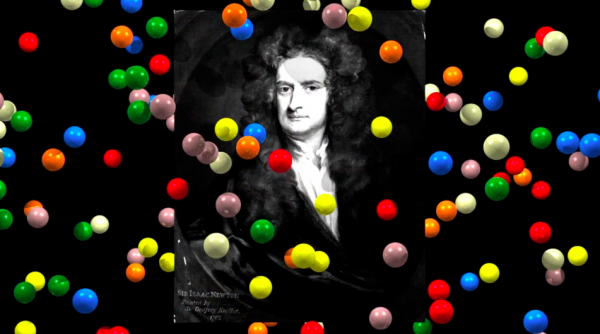The study of the solar system, with its eight planets and thousands of secondary objects is certainly not easy. What then for the atmosphere with its innumerable molecules?

Studying the trajectories of a vector field that depends on a large number of variables, this is the real challenge launched by the great Newton ...